# Learning Electronics

Learn to build electronic circuits

# Specific resistance of conductors

### Question 1:

Given two lengths of metal wire, which one will have the least electrical resistance: one that is short, or one that is long? Assume all other factors are equal (same metal type, same wire diameter, etc.).
The short wire will have less electrical resistance than the long wire.

Notes:
Many analogies exist to express this concept: water through a pipe, compressed air through a hose, etc. Which pipe or hose is less restrictive: the short one or the long one?

### Question 2:

Given two lengths of solid metal wire with round cross-sections, which one will have the least electrical resistance: one that is small-diameter, or one that is large-diameter? Assume all other factors are equal (same metal type, same wire length, etc.).
The large-diameter wire will have less electrical resistance than the small-diameter wire.

Notes:
Many analogies exist to express this concept: water through a pipe, compressed air through a hose, etc. Which pipe or hose is less restrictive: the skinny one or the fat one?

### Question 3:

What is specific resistance, symbolized by the Greek letter "rho" (r)?
Specific resistance is a measure of how resistive any particular substance is, relative to its length and cross-sectional area.

Notes:
Ask your students, "Why is it important to have a quantity called specific resistance? Why don't we just compare the "resistivity" of different substances in regular units of ohms?

### Question 4:

Write a single equation relating the resistance, specific resistance, length, and cross-sectional area of an electrical conductor together.

 R = r l A

Where,
R = Resistance, measured along the conductor's length
r = Specific resistance of the substance
l = Length of the conductor
A = Cross-sectional area of the conductor
Follow-up question: algebraically manipulate this equation to solve for length (l) instead of solving for resistance (R) as shown.

Notes:
A beneficial exercise to do with your students is to analyze this equation (and in fact, any equation) qualitatively instead of just quantitatively. Ask students what will happen to R if r increases, or if l decreases, or if A decreases. Many students find this a more challenging problem than working with real numbers, because they cannot use their calculators to give them qualitative answers (unless they enter random numbers into the equation, then change one of those numbers and re-calculate - but that is twice the work of solving the equation with one set of numbers, once!).

### Question 5:

Examine the following specific resistance table for various metals:

 Metal type r in W · cmil / ft @ 32oF r in W · cmil / ft @ 75oF Zinc (very pure) 34.595 37.957 Tin (pure) 78.489 86.748 Copper (pure annealed) 9.390 10.351 Copper (hard-drawn) 9.810 10.745 Copper (annealed) 9.590 10.505 Platinum (pure) 65.670 71.418 Silver (pure annealed) 8.831 9.674 Nickel 74.128 85.138 Steel (wire) 81.179 90.150 Iron (approx. pure) 54.529 62.643 Gold (99.9 % pure) 13.216 14.404 Aluminum (99.5 % pure) 15.219 16.758

Of the metals shown, which is the best conductor of electricity? Which is the worst? What do you notice about the resistivity of these metals as temperature is increased from 32oF to 75 oF?
Here is the same table, the order re-arranged to show resistivity from least to greatest:

 Metal type r in W · cmil / ft @ 32oF r in W · cmil / ft @ 75oF Silver (pure annealed) 8.831 9.674 Copper (pure annealed) 9.390 10.351 Copper (annealed) 9.590 10.505 Copper (hard-drawn) 9.810 10.745 Gold (99.9 % pure) 13.216 14.404 Aluminum (99.5 % pure) 15.219 16.758 Zinc (very pure) 34.595 37.957 Iron (approx. pure) 54.529 62.643 Platinum (pure) 65.670 71.418 Nickel 74.128 85.138 Tin (pure) 78.489 86.748 Steel (wire) 81.179 90.150

Notes:
The data for this table was taken from table 1-97 of the American Electrician's Handbook (eleventh edition) by Terrell Croft and Wilford Summers.
It may come as a surprise to some students to find that gold is actually a worse conductor of electricity than copper, but the data doesn't lie! Silver is actually the best, but gold is chosen for a lot of microelectronic applications because of its resistance to oxidation.

### Question 6:

What is the electrical resistance of a 12-gauge copper wire, 500 feet long, at room temperature?
Wire resistance = 0.7726 W

Notes:
Ask your students to share their sources for data: values of r, cross-sectional area, etc.

### Question 7:

A spool holds an unknown length of aluminum wire. The size of the wire is 4 AWG. Fortunately, both ends of the wire are available for contact with an ohmmeter, to measure the resistance of the entire spool. When measured, the wire's total resistance is 0.135 W. How much wire is on the spool (assuming the spool is at room temperature)?
353.51 feet

Notes:
This question illustrates another practical application of specific resistance calculations: how to determine the length of wire on a spool. The amount of resistance in this example is quite low, being a mere fraction of an ohm. Ask your students what kinds of problems they might encounter trying to measure such a low resistance with accuracy. Would the typical errors incurred in such a low-resistance measurement tend to make their calculation of length be excessive, or too low? Why?

### Question 8:

The cross-sectional dimensions of a copper "busbar" measure 8 cm by 2.5 cm. How much resistance would this busbar have, measured end-to-end, if its length is 10 meters? Assume a temperature of 20o Celsius.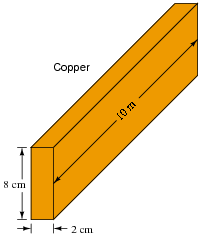83.9 mW

Notes:
This question is a good review of the metric system, relating centimeters to meters, and such. It may also be a good review of unit conversions, if students choose to do their resistance calculations using English units (cmils, or square inches) rather than metric.
Students may be surprised at the low resistance figure, but remind them that they are dealing with a solid bar of copper, over 3 square inches in cross-sectional area. This is one big conductor!

### Question 9:

Calculate the end-to-end resistance of a 20 meter length of copper wire with a diameter of 0.05 cm. Use 1.678 ×10-6  W·cm for the specific resistance of copper.
1.709 W

Notes:
Nothing to comment on here - just a straight-forward calculation of resistance. Students need to be careful of the centimeter dimension, though!

### Question 10:

Calculate the amount of power delivered to the load resistor in this circuit: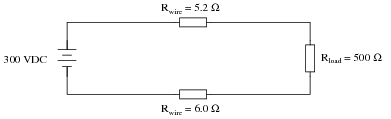Also, calculate the amount of power that would be delivered to the load resistor if the wires were superconducting (Rwire = 0.0  W).
Pload 170 Watts (with resistive wire)
Pload = 180 Watts (with superconducting wire)
Follow-up question: Compare the direction of current through all components in this circuit with the polarities of their respective voltage drops. What do you notice about the relationship between current direction and voltage polarity for the battery, versus for all the resistors? How does this relate to the identification of these components as either sources or loads?

Notes:
Not only is this question good practice for series circuit calculations (Ohm's and Joule's Laws), but it also introduces superconductors in a practical context.

### Question 11:

Suppose a power system were delivering AC power to a resistive load drawing 150 amps: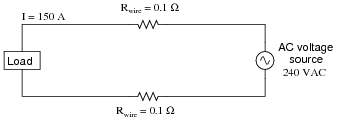Calculate the load voltage, load power dissipation, the power dissipated by the wire resistance (Rwire), and the overall power efficiency, indicated by the Greek letter ëta" (h = [(Pload)/(Psource)]).

Eload =
Pload =
Plines =
h =

Now, suppose we were to re-design both the generator and the load to operate at 2400 volts instead of 240 volts. This ten-fold increase in voltage allows just one-tenth the current to convey the same amount of power. Rather than replace all the wire with different wire, we decide to use the exact same wire as before, having the exact same resistance (0.1 W per length) as before. Re-calculate load voltage, load power, wasted power, and overall efficiency of this (higher voltage) system: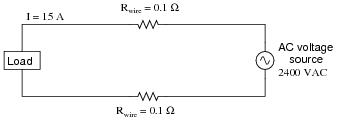Eload =
Pload =
Plines =
h =

240 volt system:
Eload = 210 volts
Pload = 31.5 kW
Plines = 4.5 kW
h = 87.5 %

2400 volt system:
Eload = 2397 volts
Pload = 35.96 kW
Plines = 45 W
h = 99.88 %

Notes:
An example like this usually does a good job clarifying the benefits of using high voltage over low voltage for transmission of large amounts of electrical power over substantial distances.

### Question 12:

The efficiency (h) of a simple power system with losses occurring over the wires is a function of circuit current, wire resistance, and total source power: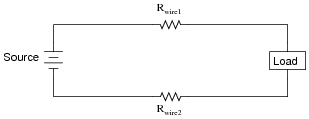A simple formula for calculating efficiency is given here:

 h = Psource - I2R Psource

Where,
Psource = the power output by the voltage source, in watts (W)
I = the circuit current, in amperes (A)
R = the total wire resistance (Rwire1 + Rwire2), in ohms (W)
Algebraically manipulate this equation to solve for wire resistance (R) in terms of all the other variables, and then calculate the maximum amount of allowable wire resistance for a power system where a source outputting 200 kW operates at a circuit current of 48 amps, at a minimum efficiency of 90%.

 R = Psource - hPsource I2

The maximum allowable (total) wire resistance is 8.681 W.

Notes:
A common mistake for students to make here is entering 90% as "90" rather than as "0.9" in their calculators.

### Question 13:

What size (gauge) of copper wire is needed in this circuit to ensure the load receives at least 110 volts?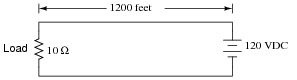#6 gauge copper wire comes close, but is not quite large enough. #5 gauge or larger will suffice.

Notes:
Several steps are necessary to solve this problem: Ohm's Law, algebraic manipulation of the specific resistance equation, and research into wire sizes. Be sure to spend adequate time discussing this problem with your students!
The concept of a generic "load" is any component or device that dissipates electrical power in a circuit. Often, generic loads are symbolized by a resistor symbol (a zig-zag line), even though they might not really be a resistor.

### Question 14:

A strain gauge is a type of sensing device widely used in the aerospace industry, for testing of vehicles and mechanical components. Explain what a strain gauge does, and how it functions.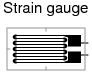A strain gauge converts micro-mechanical motions (ßtrain") into electrical resistance changes. Typically, strain gauges are used to measure the stretching, compressing, and twisting of metal components under stress.

Notes:
Ask your students to relate their answers to the illustration shown in the question. How does that strange-looking device actually measure strain? How does its resistance change, and why?

### Question 15:

How does the conductance (G) of a conductor relate to its length? In other words, the longer the conductor, the (fill-in-the-blank) its conductance is, all other factors being equal.
Conductance decreases as length increases, all other factors being equal.
Follow-up question: how does "conductance" (G) mathematically relate to resistance (R), and what is the unit of measurement for conductance?

Notes:
There are two units of measurement for conductance: the old unit (which makes perfect sense, even though your students may laugh at it, at first), and the new unit (named after a famous electrical researcher). Make sure your students are familiar with both.